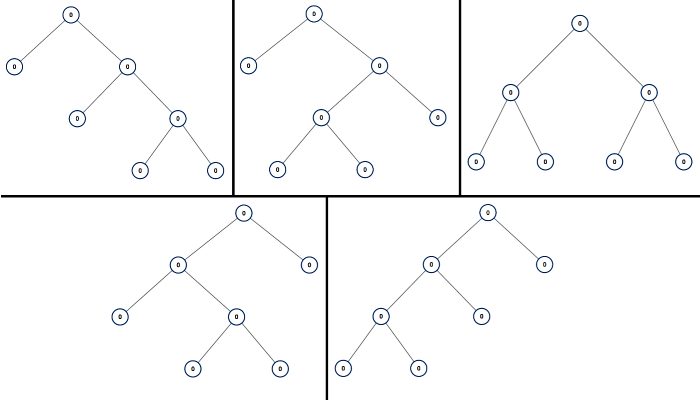# 894. All Possible Full Binary Trees

full binary tree is a binary tree where each node has exactly 0 or 2 children.

Return a list of all possible full binary trees with `N`nodes.  Each element of the answer is the root node of one possible tree.

Each `node` of each tree in the answer must have `node.val = 0`.

You may return the final list of trees in any order.

Example 1:

``````Input: 7
Output: [[0,0,0,null,null,0,0,null,null,0,0],[0,0,0,null,null,0,0,0,0],[0,0,0,0,0,0,0],[0,0,0,0,0,null,null,null,null,0,0],[0,0,0,0,0,null,null,0,0]]
Explanation:
``````Note:

• `1 <= N <= 20`

这道题给了一个数字N，让我们生成所有包含N个结点的满二叉树。所谓的满二叉树，就是每个结点一定会有0个或2两个子结点，换句话说，子结点必须成对出现，注意跟完全二叉树区分。现在我们有N个结点可以使用，若我们仔细观察，可以发现，所有的满二叉树的结点总数都是奇数，所以只要当N为偶数的时候，一定返回的是空数组，这个可以当作一个剪枝放在开头。下面我们就来考虑当N是奇数时，如何生成不同的满二叉树。先从最简单的开始，当 N=1 时，就只有一个根结点，当 N=3 时，也只有一种情况，根结点和左右子结点，当 N=5 时，就有如下两种情况：

``````0
``````

/
0 0
/
0 0

``````0
``````

/
0 0

`````` / \
0   0
``````

``````class Solution {
public:
vector<TreeNode*> allPossibleFBT(int N) {
if (N % 2 == 0) return {};
if (N == 1) return {new TreeNode(0)};
vector<TreeNode*> res;
for (int i = 1; i < N; i += 2) {
vector<TreeNode*> left = allPossibleFBT(i), right = allPossibleFBT(N - i - 1);
for (auto a : left) {
for (auto b : right) {
TreeNode *cur = new TreeNode(0);
cur->left = a;
cur->right = b;
res.push_back(cur);
}
}
}
return res;
}
};
``````

``````class Solution {
public:
unordered_map<int, vector<TreeNode*>> m;
vector<TreeNode*> allPossibleFBT(int N) {
if (N % 2 == 0) return {};
if (N == 1) return {new TreeNode(0)};
if (m.count(N)) return m[N];
vector<TreeNode*> res;
for (int i = 1; i < N; i += 2) {
vector<TreeNode*> left = allPossibleFBT(i), right = allPossibleFBT(N - i - 1);
for (auto a : left) {
for (auto b : right) {
TreeNode *cur = new TreeNode(0);
cur->left = a;
cur->right = b;
res.push_back(cur);
}
}
}
return m[N] = res;
}
};
``````

Github 同步地址:

https://github.com/grandyang/leetcode/issues/894

Unique Binary Search Trees II

 微信打赏Venmo 打赏